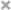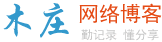# 怎么使用Canvas绘制虚线

 1 `ctx.setLineDash(segments);`

 123456789 `function` `drawDashed(){``　　cxt.lineWidth = 4;``　　cxt.strokeStyle = ``'green'``;``　　cxt.beginPath();``　　cxt.setLineDash([5, 15]);``　　cxt.moveTo(20, 20);``　　cxt.lineTo(400, 20);``　　cxt.stroke();``}`1 `= 4= ``'green'``50, 60400, 60= 4= ``'red'``0, 100400, 100`123456789101112131415161718192021222324 `function` `drawDashed(){``    ``cxt.lineWidth = 4;` `    ``cxt.strokeStyle = ``'blue'``;``    ``cxt.beginPath();``    ``cxt.setLineDash([20, 5]);``    ``cxt.moveTo(20, 40);``    ``cxt.lineTo(380, 40);``    ``cxt.stroke();` `    ``cxt.strokeStyle = ``'green'``;``    ``cxt.beginPath();``    ``cxt.setLineDash([10, 20, 30]);``    ``cxt.moveTo(20, 80);``    ``cxt.lineTo(380, 80);``    ``cxt.stroke();` `    ``cxt.strokeStyle = ``'red'``;``    ``cxt.beginPath();``    ``cxt.setLineDash([10, 20, 30, 40]);``    ``cxt.moveTo(20, 120);``    ``cxt.lineTo(380, 120);``    ``cxt.stroke();``}`getLineDash 方法

 1 `ctx.getLineDash()`

?
?

1. 获取起点坐标
2. 计算虚线的总长度，计算虚线包含多少短线然后循环绘制

 123456789101112 `var` `canvas = document.getElementById(``'canvas'``);``var` `cxt = canvas.getContext(``'2d'``);``var` `moveToFunction = CanvasRenderingContext2D.prototype.moveTo;``CanvasRenderingContext2D.prototype.moveToLocation = {};``// 重新定义moveTo方法CanvasRenderingContext2D.prototype.moveTo = function (x, y){this.moveToLocation.x = x;this.moveToLocation.y = y;``    ``moveToFunction.apply(this, [x, y]);``};``CanvasRenderingContext2D.prototype.dashedLineTo = ``function``(x, y, dashedLength){``    ``dashedLength = dashedLength === undefined ? 5 : dashedLength;``var` `startX = this.moveToLocation.x;``var` `startY = this.moveToLocation.y;``var` `deltaX = x - startX;``var` `deltaY = y - startY;``var` `numberDash = Math.``floor``(Math.sqrt(deltaX*deltaX + deltaY*deltaY)/dashedLength);``for``(``var` `i=0; i < numberDash; i++){this[i%2 === 0 ? ``'moveTo'` `: ``'lineTo'``](startX + (deltaX/numberDash)*i, startY + (deltaY/numberDash)*i); ``//等同于this.moveTo(x, y)或者 this.LineTo(x, y)    }this.moveTo(x, y); //连续绘制虚线时，起点从当前点开始};//绘制虚线cxt.lineWidth = 3;``cxt.strokeStyle = ``'green'``;``cxt.moveTo(20, 20);``cxt.dashedLineTo(200, 200);``cxt.dashedLineTo(300, 100, 10);``cxt.dashedLineTo(400, 300);``cxt.stroke();`html5 canvas 图形组合是如何实现的？附代码

html5 canvas基本绘图之绘制矩形### 评论

• 欢迎访问木庄网络博客
• 可复制：代码框内的文字。
• 方法：Ctrl+C。